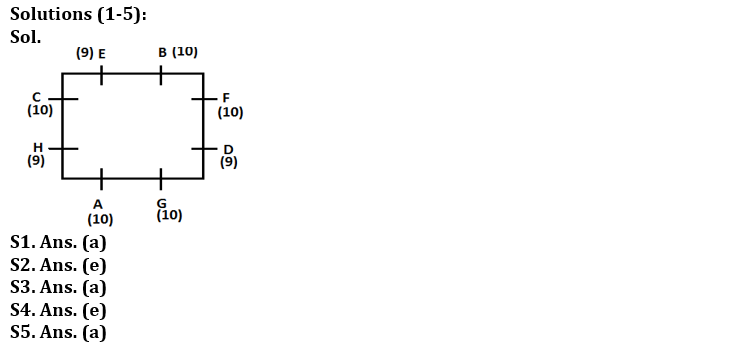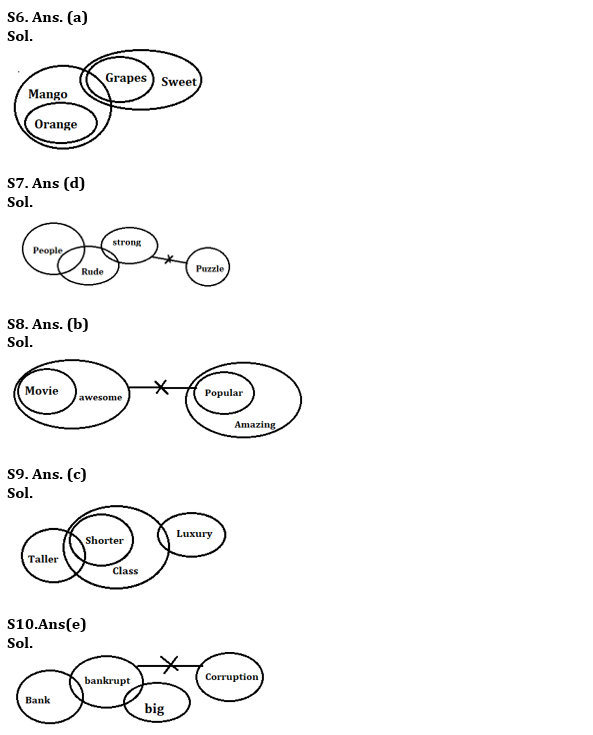Latest Banking jobs   »   Reasoning Ability Quiz For SBI Clerk...

# Reasoning Ability Quiz For SBI Clerk Prelims 2022- 22nd May

Directions (1-5): Study the following information carefully and answer the questions given below:
Eight persons sit around a square table such that two persons sit on each side of the table (facing center). Each of them either have 9 or 10 toffees. B who has 10 toffees sits 4th left to A. None of the persons having 9 toffees sit together. Not more than one person sits between H and E. Not less than three persons have same number of toffees. F faces C and both have same toffees as A. Only one person sit between F and G. G is not neighbor of B. D has odd number of toffees and sits 2nd to the right of A. E is not 3rd right to A. Two persons sit between B and H.

Q1. Who among the following has 9 toffees?
(a) E
(b) F
(c) C
(d) B
(e) None of these

Q2. Who sit 4th left to the one who is immediate right to B?
(a) E
(b) F
(c) C
(d) B
(e) None of these

Q3. How many persons sit between D and E, when counted from right of D?
(a) Two
(b) One
(c) Three
(d) Four
(e) None of these

Q4. Who among the following sit immediate right to A?
(a) E
(b) F
(c) C
(d) B
(e) None of these

Q5. Four of the following belongs to a group find the one that does not belong to that group?
(a) E
(b) F
(c) C
(d) B
(e) G

Directions (6-10): In each of the questions below are given some statements followed by some Conclusions. You have to take the given statements to be true even, if they seem to be at variance from commonly known facts. Read all the conclusions and then decide which of the given conclusions logically follows from the given statements disregarding commonly known facts.

Q6. Statements:
Only mango are orange
Only few mango are grapes
All grapes are sweet
Conclusion:
I: All mango can never be sweet
II: Some grapes can be orange

(a) If only conclusion I follows.
(b) If only conclusion II follows.
(c) If either conclusion I or II follows.
(d) If neither conclusion I nor II follows.
(e) If both conclusions I and II follow.

Q7. Statements:
Some people are rude
Some rude are Strong
No strong is puzzle
Conclusion:
I: All rude is people
II: No people is rude

(a) If only conclusion I follows.
(b) If only conclusion II follows.
(c) If either conclusion I or II follows.
(d) If neither conclusion I nor II follows.
(e) If both conclusions I and II follow.

Q8. Statements:
All movies are awesome
No awesome is popular
All popular is amazing
Conclusions:
I. Some awesome are not amazing
II. Some amazing can never be movie

(a) If only conclusion I follows.
(b) If only conclusion II follows.
(c) If either conclusion I or II follows.
(d) If neither conclusion I nor II follows.
(e) If both conclusions I and II follow.

Q9. Statements:
Some taller are shorter
All shorter are Class
Some class are luxury
Conclusions:
I. Some taller are not class
II. All taller are class

(a) If only conclusion I follows.
(b) If only conclusion II follows.
(c) If either conclusion I or II follows.
(d) If neither conclusion I nor II follows.
(e) If both conclusions I and II follow.

Q10. Statements:
Only a few bank are bankrupt
Some bankrupt are big
No bankrupt is corruption
Conclusions:
I: All bank can be Big
II: All corruption can be Big

(a) If only conclusion I follows.
(b) If only conclusion II follows.
(c) If either conclusion I or II follows.
(d) If neither conclusion I nor II follows.
(e) If both conclusions I and II follow.

Q11. If in the number 639429687, 1 is added to each of the digit which is less than five and 1 is subtracted from each of the digit which is greater than five then how many digits are repeating in the number thus formed?
(a) Two
(b) One
(c) None
(d) Three
(e) Four

Q12. The position of how many alphabets will remain unchanged if each of the alphabets in the word ‘TRANSPORT’ is arranged in alphabetical order from left to right?
(a) Four
(b) Three
(c) None
(d) One
(e) Two

Q13. Which of the following elements should come in a place ‘?’ ?
DF8 HJ12 LN16 ?
(a) PR19
(b) PR18
(c) PR21
(d) PR22
(e) None of these

Q14. Which of the following symbols should replace the sign (@) and (%) respectively in the given expression in order to make the expression B ≥ C and H > K definitely true?
B ≥ D ≥ F = E = K @ C ≤ A % H
(a) ≤, =
(b) ≤, ≤
(c) >, ≤
(d) =, <
(e) ≥, <

Q15. Which of the following will be definitely true if the given expression A≥D≥G=K<H=M<Q≤R is definitely true?
(a) A < H
(b) D > G
(c) R > K
(d) R ≥ G
(e) A < M

SolutionsS11. Ans(a)

S12.Ans(d)
Sol. TRANSPORT
ANOPRRSTT

S13. Ans(e)
Sol. PR20

S14. Ans.(d)

S15. Ans.(c)#### Congratulations!